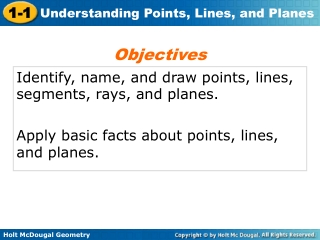DownloadDownload PresentationIdentify, name, and draw points, lines, segments, rays, and planes.

# Identify, name, and draw points, lines, segments, rays, and planes.

Download Presentation## Identify, name, and draw points, lines, segments, rays, and planes.

- - - - - - - - - - - - - - - - - - - - - - - - - - - E N D - - - - - - - - - - - - - - - - - - - - - - - - - - -
##### Presentation Transcript

1. Objectives Identify, name, and draw points, lines, segments, rays, and planes. Apply basic facts about points, lines, and planes.

2. Vocabulary undefined term point line plane collinear coplanar segment endpoint ray opposite rays postulate

3. - _______________________ The most basic figures in geometry which cannot be defined by using other figures. The undefined terms point, line, and plane are the building blocks of geometry.

4. K L M N ____________- Points that lie on the same line. K, L, and M are collinear. K, L, and N are ___________. ____________- Points that lie on the same plane. Otherwise they are ___________.

5. Example 1: Naming Points, Lines, and Planes A. Name four coplanar points. B. Name three lines.

6. Example 1C Use the diagram to name two planes.

7. Example 2: Drawing Segments and Rays Draw and label each of the following. A. a segment with endpoints M and N. B. opposite rays with a common endpoint T.

8. Example 2C Draw and label a ray with endpoint M that contains N.

9. ________, or axiom, - a statement that is accepted as true without proof. Postulates about points, lines, and planes help describe geometric properties.

10. Example 3A: Identifying Points and Lines in a Plane Name a line that passes through two points.

11. Example 3B Name a plane that contains three noncollinear points.

12. ___________ - the set of all points that two or more figures have in common.

13. Example 4: Representing Intersections A. Sketch two lines intersecting in exactly one point. B. Sketch a figure that shows a line that lies in a plane.

14. Example 4C Sketch a figure that shows two lines intersect in one point in a plane, but only one of the lines lies in the plane. Use a dashed line to show the hidden parts of any figure that you are drawing. A dashed line will indicate the part of the figure that is not seen.